# Spectral Analysis of Time Series

Load in the nino3 sea surface temperature data. These data are an average of monthly sea surface temperatures over the East Equatorial Pacific.

nino <- read.table("../Data/nino3data.asc", skip = 3)
names(nino) <- c("Year", "SST", "SSA")
plot(nino$Year, nino$SST, type = "l")

library(gridExtra)

## Loading required package: grid

library(ggplot2)
plot1 <- ggplot(data = nino) + geom_line(aes(y = SST, x = Year))
plot2 <- ggplot(data = nino) + geom_line(aes(y = SSA, x = Year))
grid.arrange(plot1, plot2)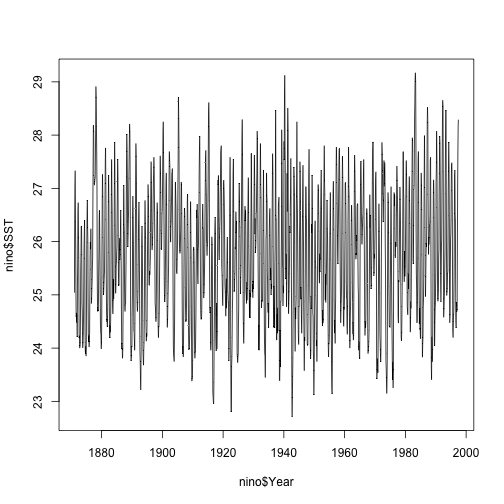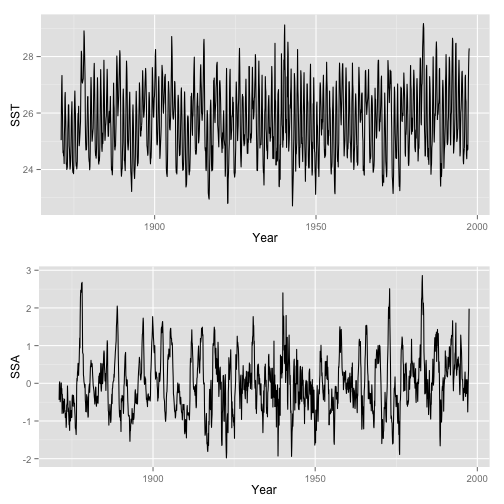## Autocorrelation plots

acf1 <- acf(nino$SST, lag.max = 12 * 20, plot = FALSE) acf2 <- acf(nino$SSA, lag.max = 12 * 20, plot = FALSE)
plot1 <- ggplot() + geom_line(aes(x = acf1$lag/12, y = acf1$acf))
plot2 <- ggplot() + geom_line(aes(x = acf2$lag/12, y = acf2$acf))
grid.arrange(plot1, plot2)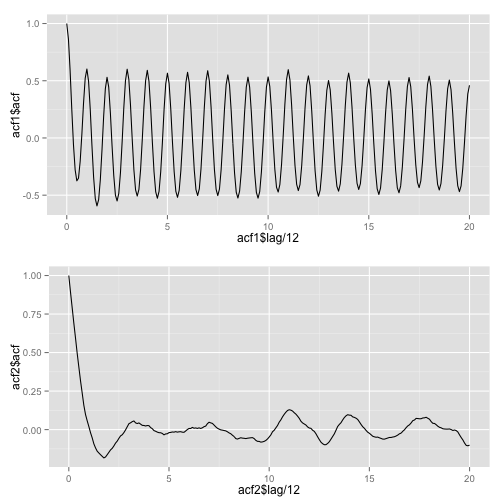## Harmonic Regression

The purpose of spectral analysis is to decompose a time series into periodic components. We might consider doing this with a regression, where we regress the time series on a set of sine and cosine waves. For a dataset with annual variation, we might expect that the sine and cosine waves with one year might be important, but what other waves might be present in this time series?

Let's try a “harmonic regression'' in which we regress the time series on harmonics (waves). I've included here annual harmonics, as well as other harmonics, some of which I think might duplicate the El Nino/La Nina cycle around 3-6 years.

# Create dataframe with different harmonics
X <- data.frame(Year=nino$Year, y = nino$SST,
sin(2*pi*1*nino$Year), cos(2*pi*1* nino$Year), # sine and cos for frequency = 1
sin(2*pi*2*nino$Year), cos(2*pi*2*nino$Year), # freq. equals 2 (i.e. period= 6 months)
sin(2*pi*1/3*nino$Year), cos(2*pi*1/3*nino$Year), # freq = 1/3 (period=3 years)
sin(2*pi*1/3.5*nino$Year), cos(2*pi*1/3.5*nino$Year), # freq=3.5 (period=3.5 years)
sin(2*pi*1/6*nino$Year), cos(2*pi*1/6*nino$Year),   # freq=6 (period=6 years)
sin(2*pi*1.01*nino$Year), cos(2*pi*1.01*nino$Year) # freq=1.01 (period=.99 years)
)
ggplot(data=subset(X, Year>1980)) + geom_line(aes(x=Year, y=X[X$Year>1980,3]))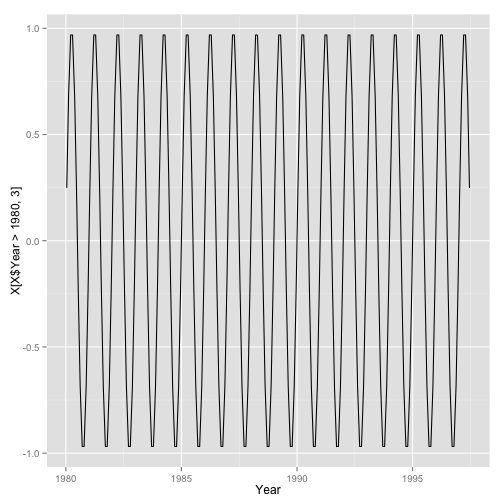ggplot(data=subset(X, Year>1980)) + geom_line(aes(x=Year, y=X[X$Year>1980,5]))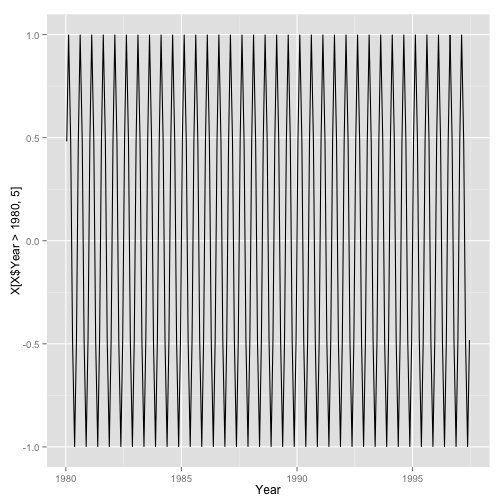ggplot(data=subset(X, Year>1980)) + geom_line(aes(x=Year, y=X[X$Year>1980,7]))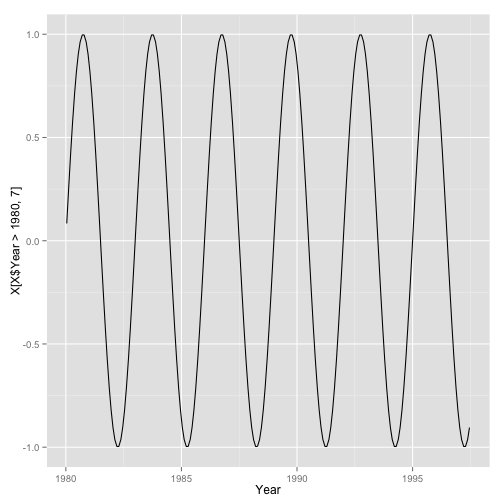ggplot(data=subset(X, Year>1980)) + geom_line(aes(x=Year, y=X[X$Year>1980,9]))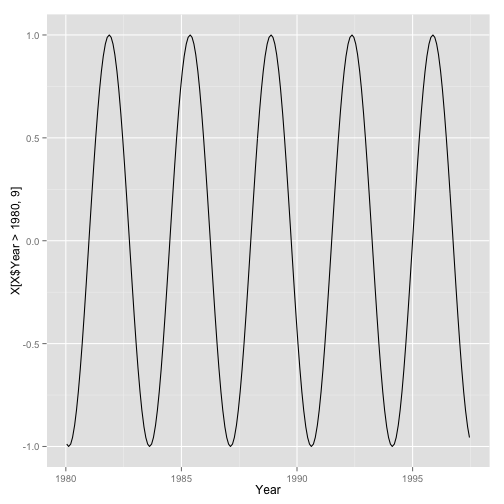ggplot(data=subset(X, Year>1980)) + geom_line(aes(x=Year, y=X[X$Year>1980,11]))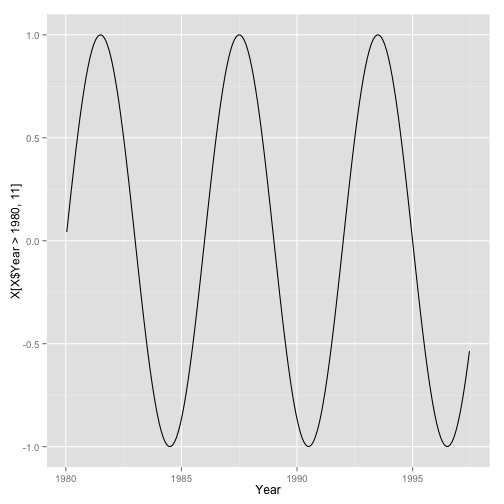Now that we've created a dataframe that has sines and cosines, we might see how well these predict the data series. We might regress SST on these sines and cosines. mod <- lm(y ~ . - Year, data = X) # Regress y on everything (but Year) summary(mod)  ## ## Call: ## lm(formula = y ~ . - Year, data = X) ## ## Residuals: ## Min 1Q Median 3Q Max ## -2.0478 -0.5220 -0.0544 0.4492 2.7313 ## ## Coefficients: ## Estimate Std. Error t value Pr(>|t|) ## (Intercept) 25.7119 0.0193 1331.89 < 2e-16 *** ## sin.2...pi...1...nino.Year. 1.2815 0.0281 45.68 < 2e-16 *** ## cos.2...pi...1...nino.Year. -0.2815 0.0275 -10.24 < 2e-16 *** ## sin.2...pi...2...nino.Year. -0.1903 0.0277 -6.88 8.7e-12 *** ## cos.2...pi...2...nino.Year. -0.2229 0.0269 -8.27 2.9e-16 *** ## sin.2...pi...1.3...nino.Year. 0.0984 0.0272 3.61 0.00031 *** ## cos.2...pi...1.3...nino.Year. -0.0625 0.0273 -2.29 0.02235 * ## sin.2...pi...1.3.5...nino.Year. -0.1047 0.0273 -3.84 0.00013 *** ## cos.2...pi...1.3.5...nino.Year. -0.1915 0.0273 -7.01 3.5e-12 *** ## sin.2...pi...1.6...nino.Year. -0.0290 0.0273 -1.06 0.28857 ## cos.2...pi...1.6...nino.Year. 0.0632 0.0273 2.32 0.02074 * ## sin.2...pi...1.01...nino.Year. -0.0296 0.0278 -1.07 0.28586 ## cos.2...pi...1.01...nino.Year. 0.0203 0.0278 0.73 0.46517 ## --- ## Signif. codes: 0 '***' 0.001 '**' 0.01 '*' 0.05 '.' 0.1 ' ' 1 ## ## Residual standard error: 0.752 on 1505 degrees of freedom ## Multiple R-squared: 0.622, Adjusted R-squared: 0.619 ## F-statistic: 206 on 12 and 1505 DF, p-value: <2e-16  What's significant? The 1 year frequency is, but not the 1.01. The twice a year frequency is. The 3, 3.5 and 6 year frequenceis are significant. I tried other "El Nino frequencies” too, but they weren't always significant. For example, 4 years was not significant. This trial and error approach is difficult! X$resid <- residuals(mod)
X$pred <- predict(mod) ggplot(data = subset(X, Year > 1970)) + geom_line(aes(x = Year, y = y)) + geom_line(aes(x = Year, y = pred), color = "red")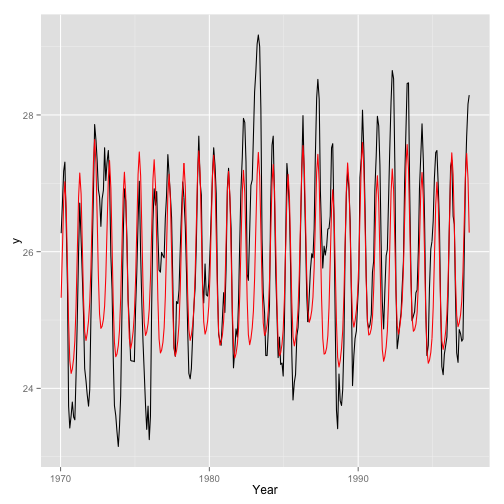We've described the annual variation pretty well. But we haven't picked up a lot of the year-to-year variation. # Frequency analysis. I could extend this regression. If I have N data, and I include N sines and cosines, then my regression will perfectly predict the data. The regression will be overfitted. But I might learn something be seeing which coefficients are significantly different from zero. This is what the “periodogram” tells us. raw.spec <- spec.pgram(nino$SST, taper = 0)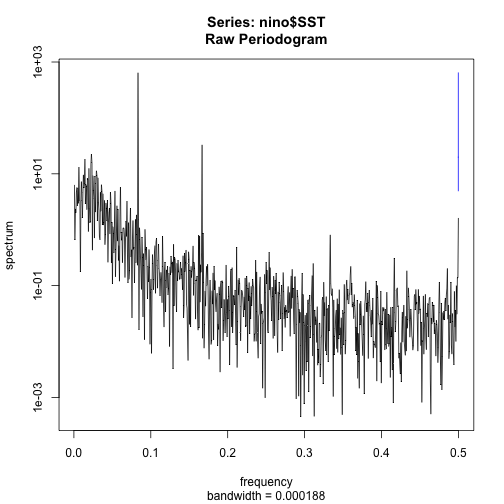plot(raw.spec)
plot(raw.spec, log = "no")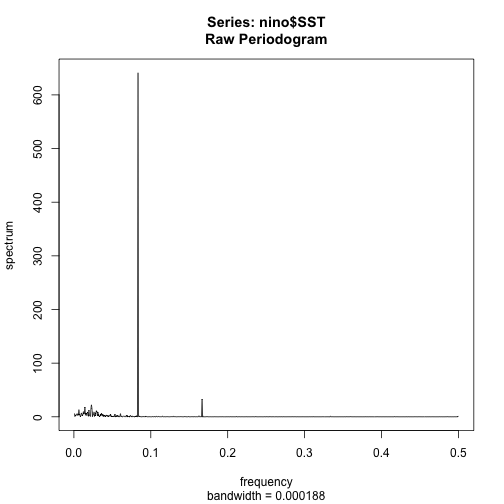# spec.df <- as.data.frame(raw.spec)
spec.df <- data.frame(freq = raw.spec$freq, spec = raw.spec$spec)
# Create a vector of periods to label on the graph, units are in years
yrs.period <- rev(c(1/6, 1/5, 1/4, 1/3, 0.5, 1, 3, 5, 10, 100))
yrs.labels <- rev(c("1/6", "1/5", "1/4", "1/3", "1/2", "1", "3", "5", "10",
"100"))
yrs.freqs <- 1/yrs.period * 1/12  #Convert annual period to annual freq, and then to monthly freq
spec.df$period <- 1/spec.df$freq
ggplot(data = subset(spec.df)) + geom_line(aes(x = freq, y = spec)) + scale_x_continuous("Period (years)",
breaks = yrs.freqs, labels = yrs.labels) + scale_y_continuous()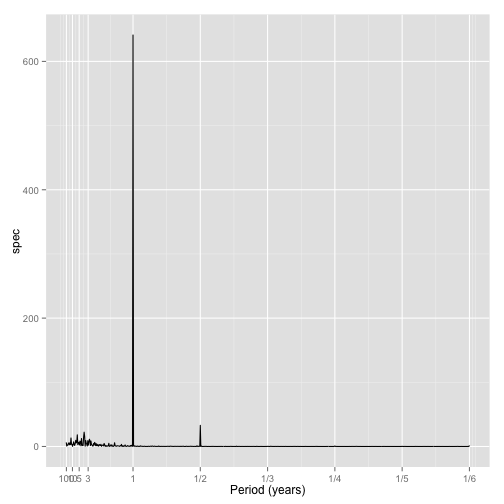Sometimes the log scaling of the spectrum is more revealing:

ggplot(data = subset(spec.df)) + geom_line(aes(x = freq, y = spec)) + scale_x_continuous("Period (years)",
breaks = yrs.freqs, labels = yrs.labels) + scale_y_log10()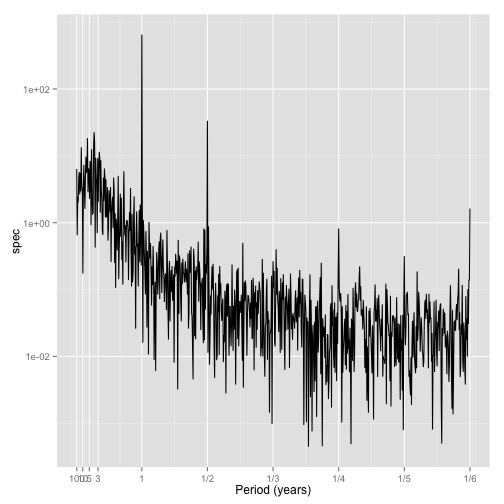The log-scaling has some theoretical advantages, too. The periodogram values should be approximately normally distributed in the log scale.

I could clean up the labels at the left end, but sometimes a log scaling can be helpful there, too, because as log scaling will spread out the low frequencies and squish the high frequencies:

ggplot(data = subset(spec.df)) + geom_line(aes(x = freq, y = spec)) + scale_x_log10("Period (years)",
breaks = yrs.freqs, labels = yrs.labels) + scale_y_log10()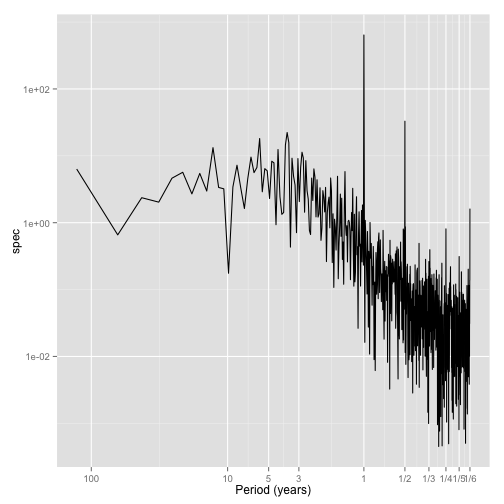Typically, it is the relatively low frequency stuff that is the most interesting. And also the most challenging to resolve. (The overlap of interesting and challenging is not mere concidence. If it were easy, we know all about it and it would stop being interesting!)

# Smoothing the periodogram:

There is a fundamental problem with the periodogram. Unlike most estimates you've encountered, such as the mean or a regression coefficient, which get more reliable as you collect more data, the periodogram does not get more reliable. As you collect more data, you add more periodogram points, but they are all just as noisy as before.

We are assuming that there is some underlying curve of spectral values, and that the periodogram estimates this. But the periodogram is noisy, and will always be noisy. We call this underlying curve the “spectral density function,” or sometimes the “power spectrum.”

The only way to get smooth estimates of the power spectrum is by taking moving averages of the periodogram. In essence, though, we want to give more weight to close frequencies, and little weight to far away frequencies. There are different ways to create weights. You could use a bell curve shape to give weights. You could use a triangle, or a rectangle. There are lots of others too. These are called 'kernel functions.'

plot(kernel("daniell", m = 10))  # A short moving average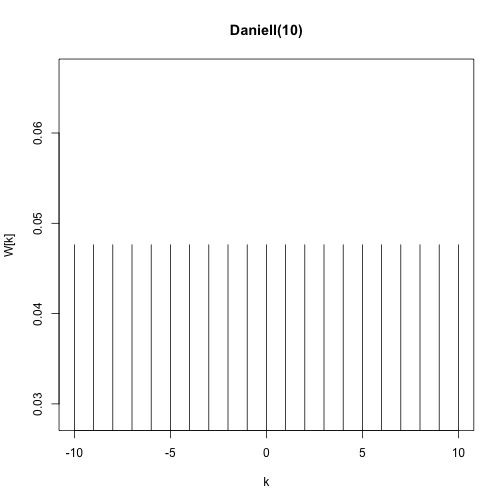plot(kernel("daniell", m = 50))  # A long moving average